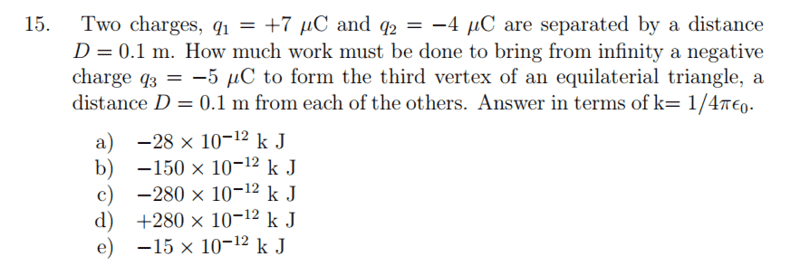# Are the answer choices wrong? (electric potential energy)

## Homework StatementU=kq1q2/r

## The Attempt at a Solution

W = changeU = Uf-Uo

Uf = k(7*(-5) + 7(-4) + (-5)*(-4))/0.1 = -4.3*10^-4
Ui= k((7*(-4))/0.1= -2.8*10^-4

Uf-Ui = -1.5*10^-4k J

#### Attachments

TSny
Homework Helper
Gold Member
Uf = k(7*(-5) + 7(-4) + (-5)*(-4))/0.1 = -4.3*10^-4
Shouldn't k appear in the expression on the right side? How did you get the power of -4? Did you take into account that the charges are in micro Coulombs? Otherwise, your approach looks right.

gneill
Mentor
The provided answer choices seem out of line for the given problem statement, but your calculated answer is also rather suspect. How did you determine the order of magnitude of the results? What value did you use for ##k##?

TSny
Homework Helper
Gold Member
Note that in the choices of answers, the symbol k represents Coulomb's constant, not kilo.

•lorx99 and gneill
The provided answer choices seem out of line for the given problem statement, but your calculated answer is also rather suspect. How did you determine the order of magnitude of the results? What value did you use for ##k##?
I aciddently left out that i multiplied by 10^-6 for the product of q's.

gneill
Mentor
I aciddently left out that i multiplied by 10^-6 for the product of q's.

I get a different result on the order of a few Joules. Maybe check your arithmetic?

•lorx99
I get a different result on the order of a few Joules. Maybe check your arithmetic?
Thanks, i entered the E-6 wrong! answer is -150e-12

gneill
Mentor
Okay, let's take a look at the initial electric potential energy of the original configuration comprised of the two first charges:

##q_1 = 7~μC##
##q_2 = -4 μC##
##D = 0.1~m##

##U_o = k\frac{q_1 q_2}{D}##
##U_o = 8.988 \times 10^9~\frac{V~m}{C}\left( \frac{7\times10^-6~C \cdot (-4\times 10^-6~C)}{0.1~m} \right)##
I find that:

##U_o = -2.52~J## or, ##U_o = -2.52~\times 10^{-3}~kJ##

So we can expect answers to be on the order of ##10^1## Joules

TSny
Homework Helper
Gold Member
##U_o = 8.988 \times 10^9~\frac{V~m}{C}\left( \frac{7\times10^-6~C \cdot (-4\times 10^-6~C)}{0.1~m} \right)##
Hi, gneill. Apparently they don't want you to substitue a value for ##k##. Thus,

##U_o = k \left( \frac{7\times10^-6~C \cdot (-4\times 10^-6~C)}{0.1~m} \right) = k~ \left(-280 \times10^{-12} \, C^2/m \right) = -280 \times10^{-12}~ k~ J##.

Here, the ##k## is Coulomb's constant (even in the final expression). The units for ##k## have been absorbed into ##J## in the last step. This is an awkward way to express the answer, but I guess they didn't want the student to bother with looking up the value of ##k##.

gneill
Mentor
Hi, gneill. Apparently they don't want you to substitue a value for ##k##. Thus,

##U_o = k \left( \frac{7\times10^-6~C \cdot (-4\times 10^-6~C)}{0.1~m} \right) = k~ \left(-280 \times10^{-12} \, C^2/m \right) = -280 \times10^{-12}~ k~ J##.

Here, the ##k## is Coulomb's constant (even in the final expression). The units for ##k## have been absorbed into ##J## in the last step. This is an awkward way to express the answer, but I guess they didn't want the student to bother with looking up the value of ##k##.
Hmm. Okay, I wasn't expecting that. When I see kJ I immediately think kilo-Joules. It seems to me a bit odd to expect students to know that they need not invoke the relevant constant values.

TSny
Homework Helper
Gold Member
Hmm. Okay, I wasn't expecting that. When I see kJ I immediately think kilo-Joules. It seems to me a bit odd to expect students to know that they need not invoke the relevant constant values.
Yes, it threw me off at first. In the problem statement, it says, "answer in terms of k = 1/(4πε0)." It could have been clearer as what was meant here.

•gneill